# Suppose

Suppose you know that the length of a line segment is 15, x2=6, y2=14 and x1= -3. Find the possible value of y1. Is there more than one possible answer? Why or why not?

Result

y11 =  26
y12 =  2

#### Solution:

$x_{2}=6 \ \\ y_{2}=14 \ \\ x_{1}=-3 \ \\ \ \\ d=15 \ \\ (x_{1}-x_{2})^2 +(y_{1}-y_{2})^2=d^2 \ \\ (-3-6)^2 +(y_{1}-14)^2=15^2 \ \\ (-3-6)^2 +(y_{1}-14)^2=15^2 \ \\ \ \\ (-3-6)^2 +(q-14)^2=15^2 \ \\ \ \\ q^2 -28q +52=0 \ \\ \ \\ a=1; b=-28; c=52 \ \\ D=b^2 - 4ac=28^2 - 4\cdot 1 \cdot 52=576 \ \\ D>0 \ \\ \ \\ q_{1,2}=\dfrac{ -b \pm \sqrt{ D } }{ 2a }=\dfrac{ 28 \pm \sqrt{ 576 } }{ 2 } \ \\ q_{1,2}=\dfrac{ 28 \pm 24 }{ 2 } \ \\ q_{1,2}=14 \pm 12 \ \\ q_{1}=26 \ \\ q_{2}=2 \ \\ \ \\ \text{ Factored form of the equation: } \ \\ (q -26) (q -2)=0 \ \\ \ \\ y_{11}=q_{1}=26$

Checkout calculation with our calculator of quadratic equations.

$y_{12}=q_{2}=2$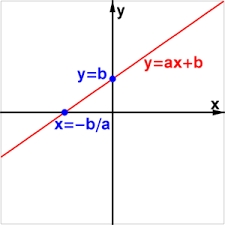Our examples were largely sent or created by pupils and students themselves. Therefore, we would be pleased if you could send us any errors you found, spelling mistakes, or rephasing the example. Thank you!

Leave us a comment of this math problem and its solution (i.e. if it is still somewhat unclear...):

Showing 1 comment:Matematik
we make circle k with centre S(x2,y2) and radius r = 15 . Then we make vertical line x= -3 . It make two intersections with circle k thus solutions are two: y11,y12.Tips to related online calculators
For Basic calculations in analytic geometry is helpful line slope calculator. From coordinates of two points in the plane it calculate slope, normal and parametric line equation(s), slope, directional angle, direction vector, the length of segment, intersections the coordinate axes etc.
Looking for help with calculating roots of a quadratic equation?
Do you have a linear equation or system of equations and looking for its solution? Or do you have quadratic equation?
Do you want to convert length units?
Pythagorean theorem is the base for the right triangle calculator.

#### You need to know the following knowledge to solve this word math problem:

We encourage you to watch this tutorial video on this math problem:

## Next similar math problems:

1. Parallels and one secant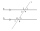There are two different parallel lines a, b and a line c that intersect the two parallel lines. Draw a circle that touches all lines at the same time.
2. Set of coordinatesConsider the following ordered pairs that represent a relation. {(–4, –7), (0, 6), (5, –3), (5, 2)} What can be concluded of the domain and range for this relation?
3. Symmetry by plane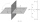Determine the coordinates of a image of point A (3, -4, -6) at a symmetry that is determined by the plane x-y-4z-13 = 0
4. Outside tangents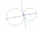Calculate the length of the line segment S1S2 if the circles k1 (S1, 8cm) and k2 (S2,4cm) touch the outside.
5. Distance problemA=(x, x) B=(1,4) Distance AB=√5, find x;
6. Two chordsIn a circle with radius r = 26 cm two parallel chords are drawn. One chord has a length t1 = 48 cm and the second has a length t2 = 20 cm, with the center lying between them. Calculate the distance of two chords.
7. Diagonal intersectisosceles trapezoid ABCD with length bases | AB | = 6 cm, CD | = 4 cm is divided into 4 triangles by the diagonals intersecting at point S. How much of the area of the trapezoid are ABS and CDS triangles?
8. Construct rhombusConstruct rhombus ABCD if given diagonal length | AC | = 8cm, inscribed circle radius r = 1.5cm
9. Three parallelsThe vertices of an equilateral triangle lie on 3 different parallel lines. The middle line is 5 m and 3 m distant from the end lines. Calculate the height of this triangle.
10. Trapezium diagonalsIt is given trapezium ABCD with bases | AB | = 12 cm, |CD| = 8 cm. Point S is the intersection of the diagonals for which |AS| is 6 cm long. Calculate the length of the full diagonal AC.
11. College 2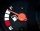College student is moving into a dormitory. The student rent a truck for $19.95 plus$0.99 per mile. Before returning the truck the student fills the tank with gasoline, which cost $65.32. Total cost$144.67. Using a linear equation, explain the process t
12. Find the 10Find the value of t if 2tx+5y-6=0 and 5x-4y+8=0 are perpendicular, parallel, what angle does each of the lines make with the x-axis, find the angle between the lines?
13. On lineOn line p: x = 4 + t, y = 3 + 2t, t is R, find point C, which has the same distance from points A [1,2] and B [-1,0].
14. Decide 2Decide whether points A[-2, -5], B[4, 3] and C[16, -1] lie on the same line
15. Three points 4The line passed through three points - see table: x y -6 4 -4 3 -2 2 Write line equation in y=mx+b form
16. Eq2 2Solve following equation with quadratic members and rational function: (x2+1)/(x-4) + (x2-1)/(x+3) = 23
17. Angle of two linesThere is a regular quadrangular pyramid ABCDV; | AB | = 4 cm; height v = 6 cm. Determine the angles of lines AD and BV.